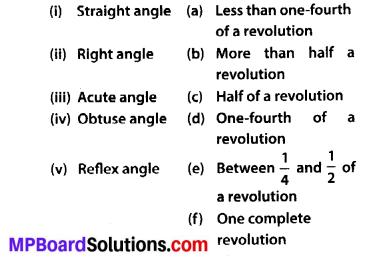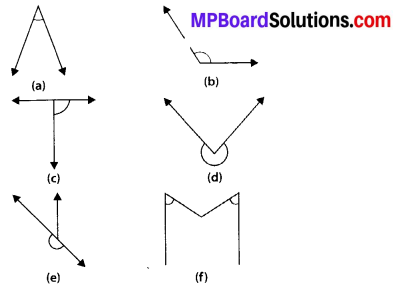# MP Board Class 6th Maths Solutions Chapter 5 Understanding Elementary Shapes Ex 5.3

## MP Board Class 6th Maths Solutions Chapter 5 Understanding Elementary Shapes Ex 5.3

Question 1.
Match the following:Solution:
(i) ➝ (c)
(ii) ➝ (d)
(iii) ➝ (a)
(iv) ➝ (e)
(v) ➝ (b)Question 2.
Classify each one of the following angles as right, straight, acute, obtuse or reflex:Solution:
(a) Acute angle
(b) Obtuse angle
(c) Right angle
(d) Reflex angle
(e) Straight angle
(f) Acute angle